# Latent Class Cluster Model in Excel tutorial

2016-05-17

This tutorial will show you how to run a Latent Class cluster model in Excel using the XLSTAT statistical software.

## Latent class cluster models: overview

In this tutorial, we use 4 categorical indicators to show how to estimate Latent Class Cluster models and interpret the resulting output. For related analyses of these data, see McCutcheon (1987), Magidson and Vermunt (2001) , and Magidson and Vermunt (2004).

In this tutorial, you will:

• Setup and estimate traditional latent class (cluster) models
• Explore which models best fit the data
• Generate and interpret output and graphs
• Obtain regression equations for scoring new cases

## Dataset for estimating Latent Class cluster models in XLSTAT

An Excel sheet containing the data for use in this tutorial can be downloaded by clicking here.

The data consists of responses from 1,202 cases on four categorical variables (PURPOSE, ACCURACY, UNDERSTA, and COOPERAT). The variable FRQ is used to denote the frequency that a specific response pattern was observed.  A sample of the data is shown in Figure 1.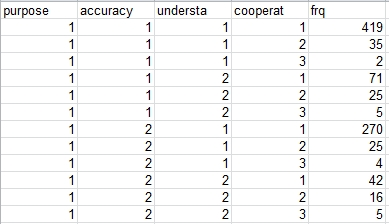Figure 1: the data (first 12 records shown)*

* Source: 1982 General Social Survey Data National Opinion Research Center

## Goal of this tutorial on Latent Class cluster models

Identify distinctly different survey respondent types (clusters) using two variables that ascertain the respondent’s opinion regarding the purpose of surveys (PURPOSE) and how accurate they are (ACCURACY), and two additional variables that are evaluations made by the interviewer of the respondent’s levels of understanding of the survey questions (UNDERSTA) and cooperation shown in answering the questions (COOPERAT). More specifically, we will focus on the criteria for choosing the number of classes (clusters), and how respondents are classified into these clusters.

## Setting up a Latent Class Cluster Model analysis in XLSTAT

To activate the XLSTAT-LatentClass cluster dialog box, select the XLSTAT / XLSTAT-LatentClass / Latent class clustering command in the Excel menu (see Figure 2).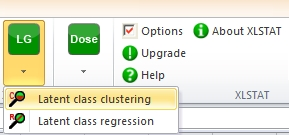Figure 2: Opening XLSTAT-LatentClass Cluster

Once you have clicked the button, the XLSTAT-LatentClass clustering dialog box is displayed.

The LC Cluster Analysis dialog box, which contains 5 tabs, opens (see Figure 3).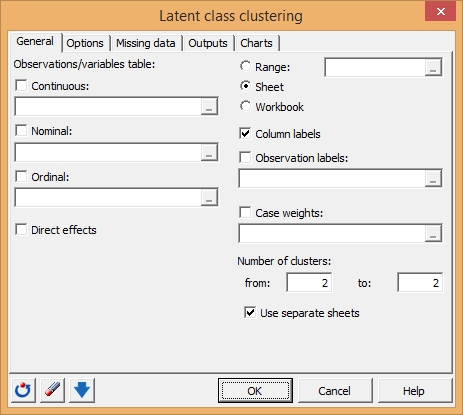Figure 3: General Tab

For this analysis, we will be using all 4 variables (PURPOSE, ACCURACY, UNDERSTA, and COOPERAT) as indicators. Since these 4 indicators are categorical variables with a small number of categories, we will use the optional case weight variable ‘FRQ’, which groups together many duplicated response patterns, reducing the size of the input data to a relatively small number of records. Alternatively we could obtain equivalent results using 1 data record for each of the 1,202 cases.

In the Observations / Nominal field, select the variables PURPOSE, ACCURACY, UNDERSTA, and COOPERAT.

In the Case weights field, select the variable FRQ.

To determine the number of clusters we will estimate 4 different cluster models, each specifying a different number of clusters. As a general rule of thumb, a good place to start is to estimate all models between 1 and 4 clusters.

Under Number of Clusters, in the box titled ‘from:’ type ‘1’ and in the box titled ‘to’ type ‘4’ to request the estimation of 4 models – a 1-cluster model, a 2-cluster model, a 3-cluster model and a 4-cluster model.

Your Dialog Box should now look like this: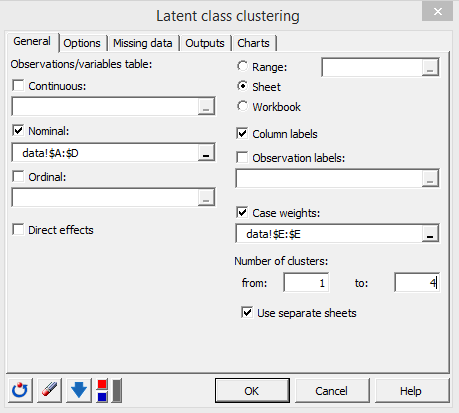Figure 4: General Tab

The fast computations start when you click on OK.

## Interpreting a Latent Class cluster analysis model output

When XLSTAT-LatentClass completes the estimation, 5 spreadsheets will be produced – a Cluster Summary sheet (Latent class clustering), and a sheet for each of the cluster models estimated (1-cluster model (LCC-1 Cluster), a 2-cluster model (LCC-2 Clusters), a 3-cluster model (LCC-3 Clusters) and a 4-cluster model (LCC-4 Clusters)).

The Latent Class Clustering summary sheet reports a summary of all the models estimated. The model L² statistic, as shown in Figure 5 in the column labeled ‘L² ’, indicates the amount of the association among the variables that remains unexplained after estimating the model; the lower the value, the better the fit of the model to the data. One criteria for determining the number of clusters is to look in the ‘p-value’ column which provides the p-value for each model under the assumption that the L² statistic follows a chi-square distribution, and select the most parsimonious model (model with the fewest number of parameters) that provides an adequate fit (p>.05). Using this criteria, the best model is given by Model 3, the 3-cluster model containing 20 parameters (p-value of 0.105).

The more general Information Criteria (BIC, AIC, AIC3) also favor parsimonious models, but this approach does not require that L² follows a chi-squared distribution, and is valid even when one or more indicators is continuous or the data is sparse due to many indicators. Using this approach we would simply select the model with the lowest value. For example, the model with the lowest BIC value is again the 3-class model (BIC=5651.121).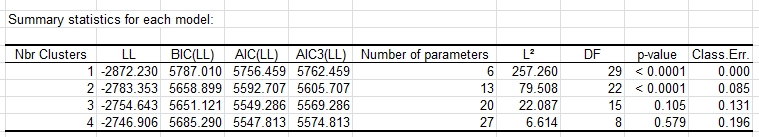Figure 5. Summary of Models Estimated

Click on the sheet ‘LCC-3 Clusters’ to view the model output for the 3-cluster model.

Following summary statistics for the 3-class model, various additional output are presented, including the Profile output in which the model parameters for each class are expressed as conditional probabilities.

Scroll down from the Summary statistics to view the Profile output (see Figure 6).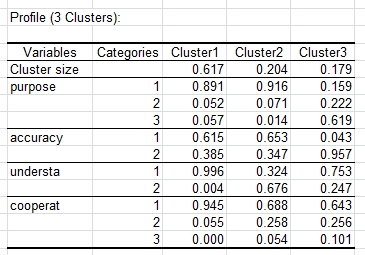Figure 6. Profile Output for 3-cluster Model

The clusters are automatically ordered according to class size. Overall, cluster 1 contains 62% of the cases, cluster 2 contains 20% and the remaining 18% are in cluster 3. The conditional probabilities show the differences in response patterns that distinguish the clusters. For example, cluster 3 is much more likely to respond that surveys are a waste of time (PURPOSE = ‘3’  / PURPOSE = ‘waste’) and that survey results are not true (ACCURACY = ‘2’ / ACCURACY = ‘not true’) than the other 2 clusters. To view these probabilities graphically, scroll down to the Profile Plot.

The Profile Plot for the 3-cluster model is shown.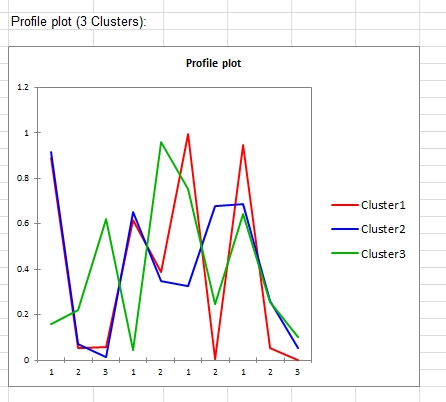Figure 7: Profile Plot for 3-cluster Model

## Classifying Cases into Clusters using Modal Assignment

Scroll down to view the Classification output: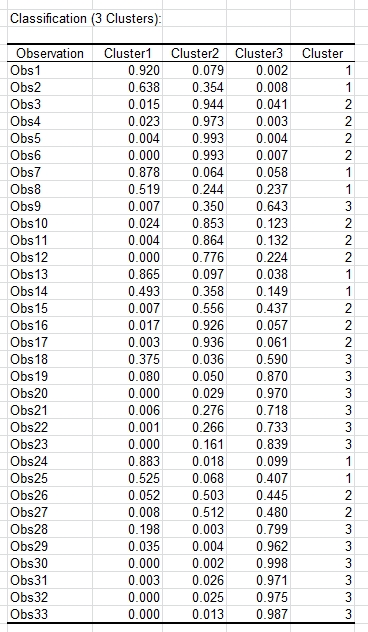Figure 8: Classification output for 3-cluster Model

The first row of the Classification Output shows that Obs1, representing all cases with the response pattern (PURPOSE = good/1, ACCURACY =mostly true/1, UNDERSTA = good/1, and COOPERAT = good/1) is classified into Cluster 1 because the probability of being in this class is highest (.920). In the column labeled ‘Cluster’, Obs1 is given the value ‘1’ indicating assignment to cluster ‘1’.

Notice that when cases are classified into clusters using the modal assignment rule, a certain amount of misclassification error is present. The expected misclassification error can be computed by cross-classifying the modal classes by the actual probabilistic classes. This is done in the Classification Table, shown in Figure 9 for the 3-class model. For this model, the modal assignment rule would be expected to classify correctly 704.0219 cases from the true cluster 1, 163.8089 from cluster 2 and 176.2545 from cluster 3 for an expected total of 1,044.085 correct classifications of the 1,202 cases. This represents an expected misclassification rate of 13.13% [(1 - 1,044.085)/1,202].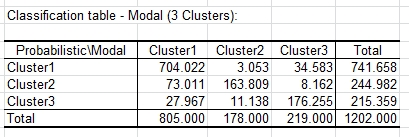Figure 9: Classification table for 3-cluster Model

Notice also that the expected sizes of the clusters are never reproduced perfectly by modal assignment. The Classification Table in Figure 9 shows that 67.0% of the total cases (805 of the 1,202) are assigned to cluster 1 using modal assignment compared to 61.7% expected to be in this cluster. (If cases were assigned to the clusters proportionately to their membership probabilities 61.7% would be expected to be classified into cluster 1).

## Interpreting bivariate residuals in latent class cluster models

In addition to various global measures of model fit, local measures called bivariate residuals are also available to assess the extent to which the 2-way association(s) between any pair of indicators are explained by the model.

Scroll down to view the Bivariate residuals output: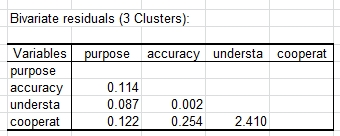Figure 10: Bivariate Residuals Output for the 3-cluster Model

The BVR corresponds to a Pearson chi-squared divided by the degrees of freedom (DF). The chi-square is computed on the observed counts in a 2-way table using the estimated expected counts obtained from the estimated model. Since the expected value of chi-squared under the assumption that the model assumptions are correct turns out to equal the degrees of freedom, if the model were true, BVRs should not be substantially larger than 1. The BVR of 2.4 in Figure 10 above suggests that the 3-cluster model may fall slightly short in reproducing the association between COOPERATE and UNDERSTAND.

In contrast, the BVRs associated with 4-cluster model (shown below in Figure 11) are all less than 1. This suggests that the 4-cluster model may provide a significant improvement over the 3-cluster model in model fit. Thus, both the 3- and 4-cluster solutions could be justified, the 3-cluster solution by BIC and the 4-cluster solution by the BVRs.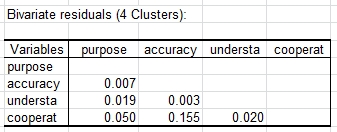Figure 11: Bivariate Residuals Output for the 4-cluster Model

## Interpreting the scoring equation

We can use the Scoring equation output to obtain regression equations for scoring new cases.

Scroll down to view the Scoring equation output: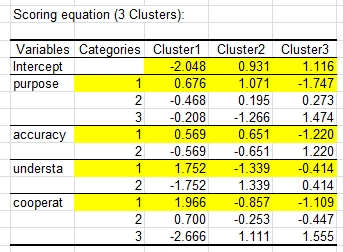Figure 12: Scoring equation Output for the 3-cluster Model

Each response pattern is scored on each cluster, and is assigned to the cluster with the highest score. For example, cases with the Obs1 response pattern:

Purpose = 1, Accuracy = 1, Understa = 1, Cooperat = 1

can be scored based on the coefficients highlighted above in yellow. This results in the following logit scores:

Cluster 1 score = 2.916, Cluster 2 score = 0.457, Cluster 3 score = -3.373.

Thus, this response pattern is assigned to Cluster 1, the cluster with the highest logit score. To obtain more meaningful scores, we can generate the posterior membership probabilities that were shown in the Classification output above using the formula provided below. This yields the following probabilities associated with the Obs1 response pattern:

Probability 1 = 0.9196, Probability 2 = 0.0787, Probability 3 = 0.0017
The formula that was used to convert the logit scores to probabilities is:

Probability (k) = exp[score(k)]/ [ exp(score1) + exp(score2) + exp(score3)]   k=1,2,3.

#### Contact our technical support team: support@xlstat.com

https://cdn.desk.com/
false
desk